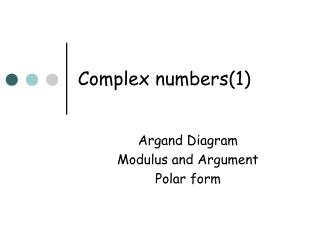DownloadDownload PresentationComplex numbers(1)

Complex numbers(1)

Download PresentationComplex numbers(1)

- - - - - - - - - - - - - - - - - - - - - - - - - - - E N D - - - - - - - - - - - - - - - - - - - - - - - - - - -
Presentation Transcript

1. Complex numbers(1) Argand Diagram Modulus and Argument Polar form

2. Complex numbers can be shown Geometrically on an Argand diagram The real part of the number is represented on the x-axis and the imaginary part on the y. -3 -4i 3 + 2i 2 – 2i Argand Diagram Im Re

3. Modulus of a complex number • A complex number can be represented by the position vector. • The Modulus of a complex number is the distance from the origin to the point. • Can you generalise this? • |z| = √(x2+y2) Im y x Re How many complex numbers in the form a + bi can you find with integer values of a and b that share the same modulus as the number above. Could you mark all of the points? What familiar shape would you draw?(more of LOCI later!)

4. Modulus questions • Find • a) |3 + 4i| = 5 • b) |5 – 12i| = 13 • c) |6 – 8i| = 10 • d) |-24 – 10i| = 26 Find the distance between the first two complex numbers above. It may help to sketch a diagram

5. The argument of a complex number a + bi No, you shut up! Shut up! No, you shut up! No, you shut up! No, you shut up!

6. Im r y θ x Re The argument of a complex number The argument of a complex number is the angle the line makes with the positive x-axis. Can you generalise this? It is really important that you sketch a diagram before working out the argument!!

7. The argument of a complex number • Calculate the modulus and argument of the following complex numbers. (Hint, it helps to draw a diagram) • 1) 3 + 4i |z| = √(32+42) = 5 • arg z = inv tan (4/3) • = 0.927 • 2) 5 – 5i |z| = √(52+52) = 5√2 • arg z = inv tan (5/-5) • = -π/4 • 3) -2√3 + 2i |z| = √((2√3)2+22) = 4 • arg z = inv tan (2/-2√3) • = 5π/6

8. The Polar form of a complex number • So far we have plotted the position of a complex number on the Argand diagram by going horizontally on the real axis and vertically on the imaginary. • This is just like plotting co-ordinates on an x,y axis • However it is also possible to locate the position of a complex number by the distance travelled from the origin (pole), and the angle turned through from the positive x-axis. • These are called “Polar coordinates”

9. (x,y) The Polar form of a complex number The ARGUMENT r is the MODULUS (r, θ) cosθ = x/r, sinθ = y/r x = r cosθ, y = r sinθ, IMAGINARY part REAL Part Im Im r y θ x Re Re

10. Converting from Cartesian to Polar • Convert the following from Cartesian to Polar • i) (1,1) = (√2,π/4) • ii) (-√3,1) = (2,5π/6) • iii) (-4,-4√3) = (8,-2π/3) Im r y θ x Re

11. Converting from Polar to Cartesian • Convert the following from Polar to Cartesian • i) (4,π/3) = (2,2√3) • ii) (3√2,-π/4) = (3,-3) • iii) (6√2,3π/4) = (-6,6) Im r y θ x Re